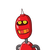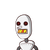# students are marching in a parade.There are 3 cadets in a row.What is the rule which gives the number of cadets,given the number o

students are marching in a parade.There are 3 cadets in a row.What is the rule which gives the number of cadets,given the number of rows?​

### 2 thoughts on “students are marching in a parade.There are 3 cadets in a row.What is the rule which gives the number of cadets,given the number o”

1.xkgxgksjfstkdtksgkgkdydkstk

Step-by-step explanation:

nxbjjdhchgchxmzgg

2.Step-by-step explanation:

We know that

No. of cadets in 1 row=3

No. of cadets in 2 row=3×2=6

No. of cadets in 3 row=3×3=9

So,

No. of cadets =3×no. of rows

Now, taking no. of rows=n

Therefore,